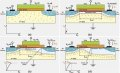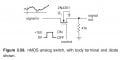# FET analog switch example of "The Art Of Electronics"

#### cake4all

Joined Oct 19, 2018
8
Hello,
I am currently reading the book "The Art of Electronics 3rd Edition". However I am having some difficulties to understand chapter "3.4.1 FET analog switch". This section is about how MOSFETs can be used for switching analog signals. As an example the attached circuit diagram (Fig. 3.59) is used.1. Source and drain of the MOSFET are not tagged. It seems as if the "signal in"-terminal can be drain as well as source. This makes it difficult for me to analyse/understand the circuit behaviour.

2. In nearly all examples the substrate and the source are shorted. In this case however, the substrate is directly connected to ground. Normally the Gate-Source voltage $$V_{GS}$$ controls the current flow $$I_D$$. But does that still hold in this case? As far as I know, the Gate-substrate voltage $$V_{GB}$$ controls how many charge carriers can flow under the gate (Inversion, see second attached image). This is due to the electric field which attracts minority charge carriers of the substrate to the gate so that a conducting channel is formed. Normally the gate-substrate voltage is equal to the gate-source voltage because they are shorted. But in this case, they are not equal. Therefore I suggest, that the current flow is not controlled by $$V_{GS}$$ instead $$V_{GB}$$ should be the important voltage. But am I right here? Most google results suggest that my opinion is wrong, however I don't understand the exact reason.The authors write a few statements that I do not understand. Maybe due to the preceding understanding problems.

1. "the gate signal is not at all critical, as long as it is sufficiently more positive than the largest signal (to maintain $$R_{On}$$ low)"

Why should the magnitude of the signal matter? If the $$V_{GB}$$ voltage controls the channel, the gate-drain voltage should be irrelevant. Maybe the authors want to point out that the FET should be in the linear region (low $$V_{DS}$$ voltage drop) for a small $$R_{On}$$? However, I have not been able to derive a formula for $$V_{DS}$$ from the given circuit diagram. Therefore, I don't understand how $$V_{DS}$$ and the signal voltage are connected.

2. "negative signals would cause the FET to turn on with the gate grounded"

Why should that be the case? Of course, negative signal voltages make the body diode between the "signal in"-terminal and the substrate conducting. That is definitely undesirable. But I don't see why negative signal voltages should somehow lead to an inversion effect in the semiconductor under the gate. So it shouldn't turn on as far as I think.

I would be grateful if somebody could help me with this issueBest wishes and merry christmasPS: I have already asked this question here. Sorry for cross posting this question on two boards, but the present answers are not very helpful for me... Maybe someone in this forum knows a good explanation who wouldn't browse on Stack Overflow.

Last edited:

#### Wolframore

Joined Jan 21, 2019
2,590Off state: gate is at ground or negative. Base at ground the Vgs stops conduction because “signal side” the gate is negatively biased for enhancement NMOS. “Signal out” side is at Ground and no conduction. If one of the sides went negative it would conduct because gate would be positive compared to it. (don’t care which side is D or S)

On state: gate is now at +15v. If this Is higher than “signal out” it would conduct... if this 15v is higher than “signal in“ side, then again the mosfet conducts. The point is it doesn’t matter which side is D or S. Gate must be higher than highest signal voltage to maintain on state.

there’s nothing to calculate except for the gate to signal potential. Higher the difference, better the conduction.

this is a switch, it does not amplify, invert just turns it on or off based on the gate and signal potential.

unless you have access to a high gate voltage relative to the signal, it may or may not be useful. Of course as you pointed out there can be nonlinearity issues

hope this helps

•cake4all

#### cake4all

Joined Oct 19, 2018
8
Thanks for your answer! Probably I am thinking far too complicated, but I really want to understand it exactly.

1) Voltage between Source and bulk?

In this lecture (http://web.mit.edu/6.012/www/SP07-L9.pdf) it is written (slide 9 to 11) that a source-bulk voltage $$V_{SB}$$ changes the threshold voltage $$V_{th}$$ of the transistor. However in this case (Fig. 3.59) the source-bulk voltage does not seem to be fixed. So for different signal voltages the threshold voltage changes. Am I correct?

2) Controlling the transistor without V_DS?

In this lecture (http://web.mit.edu/6.012/www/SP07-L7.pdf) it is written (slide 12) that the gate-bulk voltage $$V_{GB}$$ controls the inversion below the gate. Normally source and bulk are shorted so that $$V_{GB} = V_{GS}$$. This is here not the case. So why can I not simply use $$V_{GB}$$ here to control the electrical switch? My idea:
$$V_{GB} >= V_{th}$$: Transistor conducts
$$V_{GB} < V_{th}$$: Transistor does not conduct

It seems so much easier and one does not need to take care of $$V_{GS}$$ and $$V_{GD}$$.

#### Wolframore

Joined Jan 21, 2019
2,590
For the N channel mosfet, a bulk voltage higher than drain or source is not allowed, forward bias the diode junction and current would flow from bulk to the lower potential when it’s above Vfd. It must be at the lowest potential available and in this case being at ground would make it the same as source when its off and lower when MOSFET is conducting. It affects the pinch off and threshold. The bulk is normally tied to source to reduce this body effect. It would have the effect of raising Vth by the equation in your reference.

•cake4all

#### BobTPH

Joined Jun 5, 2013
6,519
That is not a MOSFET, it is a JFET.

Bob

•cake4all

#### Wolframore

Joined Jan 21, 2019
2,590
Last edited:
•cake4all